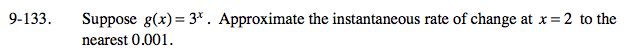### Home > PC > Chapter 9 > Lesson 9.3.3 > Problem9-133

9-133.

Suppose g(x) = 3x . Approximate the instantaneous rate of change at x = 2 to the nearest 0.001. Homework Help ✎$\lim_{h\to 0} \frac{g(2+h)-g(2)}{(2+h)-2} =\frac{3^{2+h}-3^2}{h}$

Use a calculator to approximate the IROC.